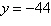Name:    Chapter 8 Pre Test

Multiple Choice
Identify the choice that best completes the statement or answers the question.

1.

Write the system of linear equations represented by the augmented matrix. (Use variables x, y, z, and w.)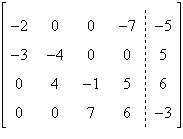a.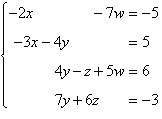b.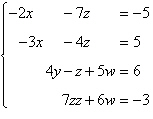c.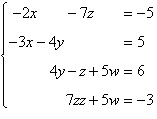d.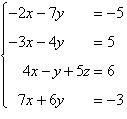e.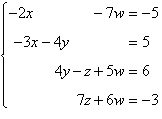2.

Identify the elementary row operation being performed to obtain the new row-equivalent matrix.
 Original Matrix New Row-Equivalent Matrix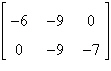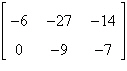a. Add –2 times R2 to R1. b. Add 2 times R2 to R1. c. Add –2 times R1 to R2. d. Add 2 times R1 to R2. e. Add 2 times R1 to R1.

3.

Write the system of linear equations represented by the augmented matrix. Then use back-substitution to solve. (Use variables x, y, and z.)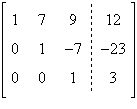a. x = 83, y = –5, z = –4 b. x = –2, y = –1, z = 3 c. x = –1, y = 2, z = –3 d. x = –1, y = –2, z = 3 e. x = 2, y = 3, z = 1

4.

Determine whether the two systems of linear equations yield the same solutions. If so, find the solutions using matrices.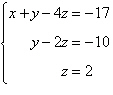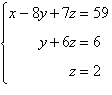a. The systems yield different solutions. b. x = 3, y = 6, z = 2 c. x = –6, y = 2, z = –3 d. x = –3, y = –6, z = 2 e. x = 3, y = 2, z = –3

5.

The currents in an electrical network are given by the solutions of the system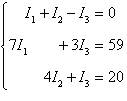where I1, I2, and I3 are measured in amperes. Solve the system of equations using matrices.
 a. I1 = 3, I2 = 5, I3 = 8 b. I1 = 5, I2 = 3, I3 = 8 c. no solution d. I1 = 5, I2 = 8, I3 = 3 e. I1 = 3, I2 = 5, I3 = 9

6.

Evaluate the expression.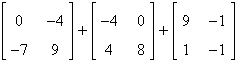a. not possible b.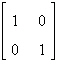c.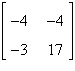d.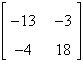e.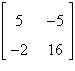7.

Evaluate the expression.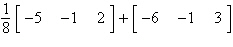a.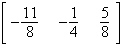b. not possible c.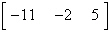d.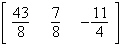e.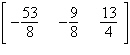8.

If possible, find AB.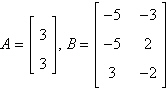a.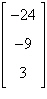b. not possible c.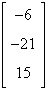d.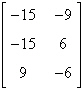e.9.

Use the matrix capabilities of a graphing utility to find AB, if possible.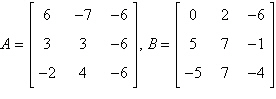a. not possible b.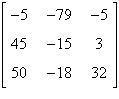c.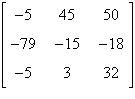d.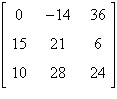e.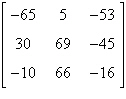10.

Find the inverse of the matrix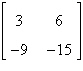.
 a.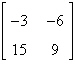b.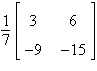c.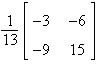d.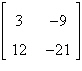e.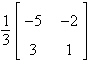11.

Find the inverse of the matrix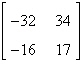(if it exists).
 a.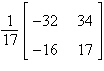b.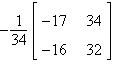c.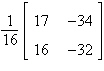d.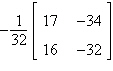e. does not exist

12.

Solve the system of linear equations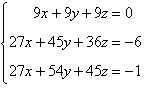using the inverse matrix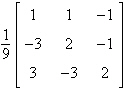.
 a.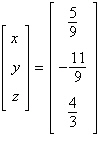b.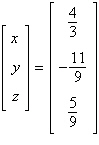c.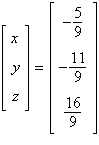d.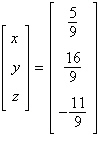e.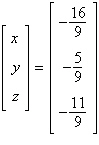13.

Solve the system of linear equations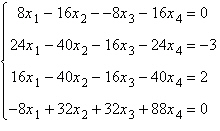using the inverse matrix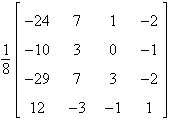.
 a.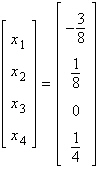d.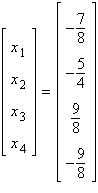b.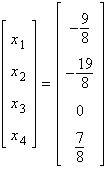e.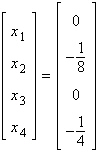c.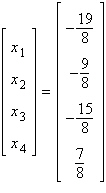14.

Solve the system of linear equations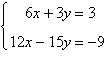using an inverse matrix.
 a.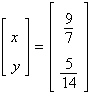b.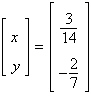c.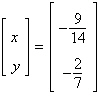d.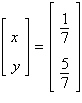e.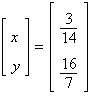15.

Find the determinant of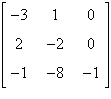.
 a. 7 b. –4 c.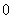d. 8 e. –3

16.

Use the matrix capabilities of a graphing utility to find the determinant of the matrix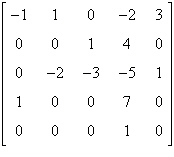.
 a. 4 b. –12 c. 7 d. –10 e. –6

17.

Use the matrix capabilities of a graphing utility to find the determinant of the matrix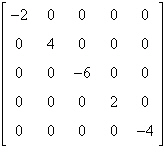.
 a. –384 b. –32 c. –64 d. –48 e. 128

18.

Given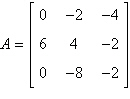, find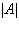.
 a. 88 b. –120 c. 168 d. 40 e. –80

19.

Solve for x given the following equation involving a determinant.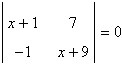a.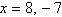b.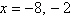c.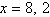d.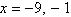e.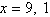20.

Use a determinant to find y such that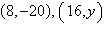, and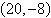are collinear.
 a.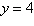b.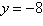c.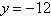d.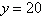e.